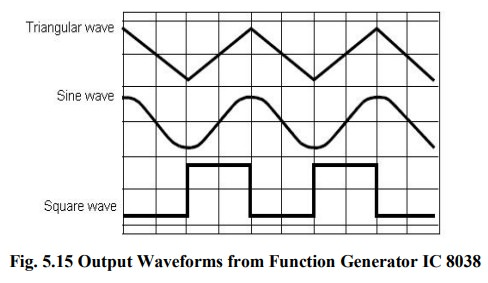Home | | Linear Integrated Circuits | Function Generator IC 8038

# Function Generator IC 8038

It consists of two current sources, two comparators, two buffers, one FF and a sine wave converter.

Function Generator IC 8038:It consists of two current sources, two comparators, two buffers, one FF and a sine wave converter.

## Pin description:

Â·           Pin 1 & Pin 12: Sine wave adjusts:

The distortion in the sine wave output can be reduced by adjusting the 100KÎ© pots connected between pin12 & pin11 and between pin 1 & 6.

Â·           Pin 2 Sine Wave Output:

Sine wave output is available at this pin. The amplitude of this sine wave is 0.22 Vcc. Where Â± 5V â‰¤ Vcc â‰¤ Â± 15 V.

Â·           Pin 3 Triangular Wave output:

Triangular wave is available at this pin. The amplitude of the triangular wave is 0.33Vcc.

Where Â± 5V â‰¤ Vcc â‰¤ Â± 15 V.

Â·           Pin 4 & Pin 5 Duty cycle / Frequency adjust:

The symmetry of all the output wave forms & 50% duty cycle for the square wave output is adjusted by the external resistors connected from Vcc to pin 4. These external resistors & capacitors at pin 10 will decide the frequency of the output wave forms.

Â·           Pin 6 + Vcc:

Positive supply voltage the value of which is between 10 & 30V is applied to this pin.

Â·           Pin 7 : FM Bias:

This pin along with pin no8 is used to TEST the IC 8038.

Â·           Pin9 : Square Wave Output:

A square wave output is available at this pin. It is an open collector output so that this pin

can be connected through the load to different power supply voltages. This arrangement is very useful in making the square wave output.

Â·           Pin 10 : Timing Capacitors:

The external capacitor C connected to this pin will decide the output frequency along with the resistors connected to pin 4 & 5.

Â·           Pin 11 : -VEE or Ground:

If a single polarity supply is to be used then this pin is connected to supply ground & if (Â±) supply voltages are to be used then (-) supply is connected to this pin.

Â·           Pin 13 & Pin 14: NC (No Connection)

### Important features of IC 8038:

1.   All the outputs are simultaneously available.

2.   Frequency range : 0.001Hz to 500kHz

3.   Low distortion in the output wave forms.

4.   Low frequency drifts due to change in temperature.

5.   Easy to use.

### Parameters:

(i)   Frequency of the output wave form:

The output frequency dependent on the values of resistors R1 & R2 along with the external capacitor C connected at pin 10.

If RA= RB = R & if RC is adjusted for 50% duty cycle then f0= 0.3/RC; RA = R1, RB =

R3, RC= R2.

(ii)  Duty cycle / Frequency Adjust : (Pin 4 & 5):

Duty cycle as well as the frequency of the output wave form can be adjusted by external resistors at pin 4 & 5.

The values of resistors RA & RB connected between Vcc pin 4 & 5 respectively along

with the capacitor connected at pin 10 decide the frequency of the wave form. The values of RA & RB should be in the range of 1kÎ© to 1MÎ©.

(iii)FM Bias:

â€˘     The FM Bias input (pin7) corresponds to the junction of resistors R1 & R2.

â€˘     The voltage Vin is the voltage between Vcc & pin8 and it decides the output frequency.

â€˘     The output frequency is proportional to Vin as given by the following expression.

For RA = RB (50% duty cycle).

f0= 5 Vin/CRAVcc ; where C is the timing capacitor.

Â·           With pin 7 & 8 connected to each other the output frequency is given by f0= 0.3/RC where R = RA = RB for 50% duty cycle.

Â·           This is because M Sweep input (pin 8):

â€˘     Vin=R1Vcc/R1+R2

â€˘     This input should be connected to pin 7, if we want a constant output frequency.

â€˘     But if the output frequency is supposed to vary, then a variable dc voltage should be applied to this pin.

â€˘     The voltage between Vcc & pin 8 is called Vin and it decides the output frequency as,

f0=1.5 Vin/CRAVCC

A potentiometer can be connected to this pin to obtain the required variable voltage required to change the output frequency.

Tags : Functional block diagram, Output waveform, Pin description, Important features, Parameters , Linear Integrated Circuits : Waveform Generators and Special Function ICs
Study Material, Lecturing Notes, Assignment, Reference, Wiki description explanation, brief detail
Linear Integrated Circuits : Waveform Generators and Special Function ICs : Function Generator IC 8038 | Functional block diagram, Output waveform, Pin description, Important features, Parameters